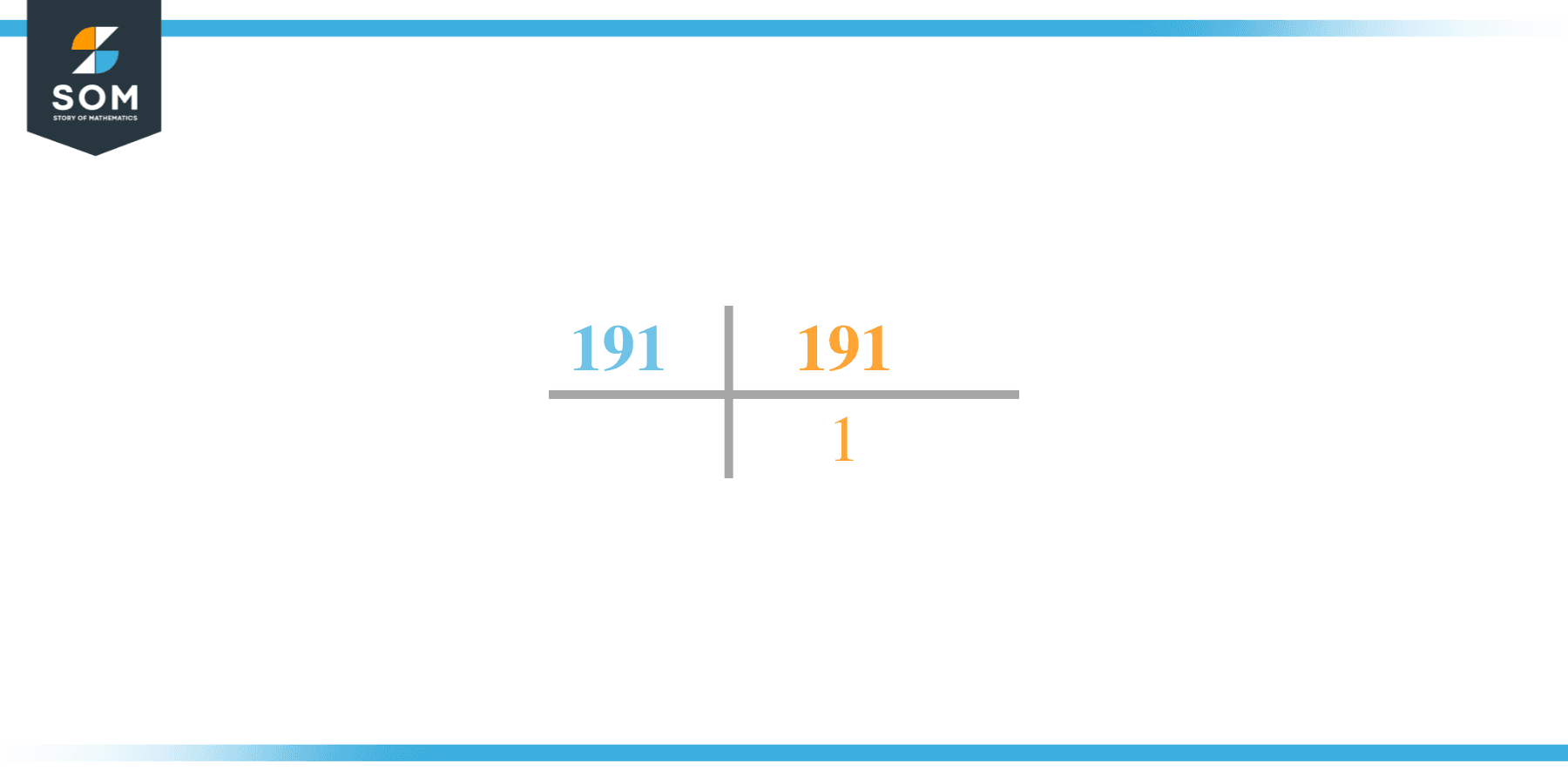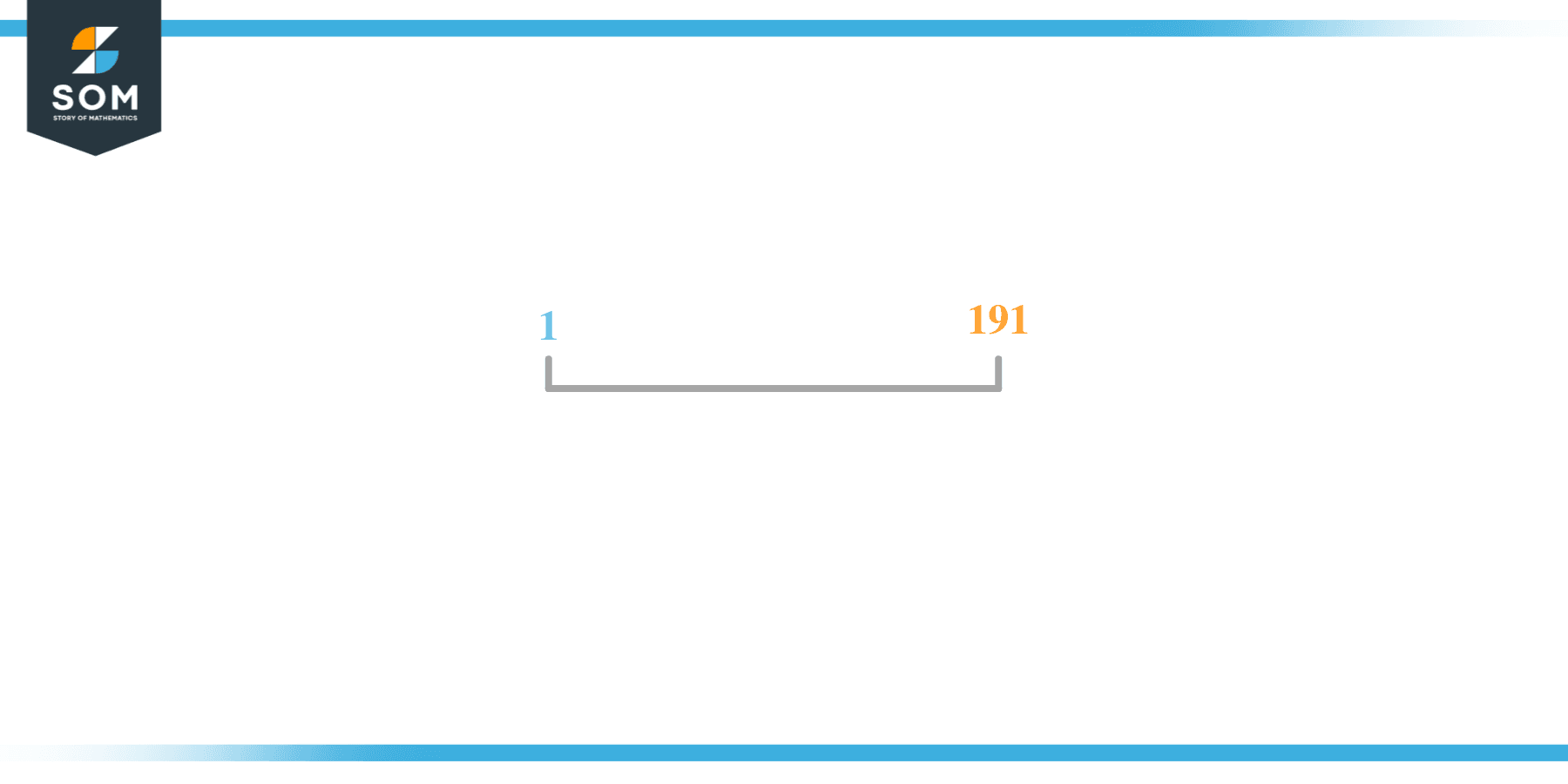# Factors of 191: Prime Factorization, Methods, and Examples

The factors of 191 are the list of those numbers that when divided by 191 leave nothing in the remainder. It is a prime number which means it can have only two factors that is 1 and the number 191 itself.The factors of the given odd number can be positive as well as negative provided that the product of any two factors is equal to the number.

### Factors of 191

Here are the factors of number 191.

Factors of 191: 1, 191

### Negative Factors of 191

The negative factors of 191 are similar to its positive factors, just with a negative sign.

Negative Factors of 191: -1, and -91

### Prime Factorization of 191

The prime factorization of 191 is the way of expressing its prime factors in the product form.

Prime Factorization: 1 x 191

In this article, we will learn about the factors of 191 and how to find them using various techniques such as upside-down division, prime factorization, and factor tree.

## What Are the Factors of 191?

The factors of 191 are 1, and 191. All of these numbers are the factors as they do not leave any remainder when divided by 191.

The factors of 191 are classified as prime numbers and composite numbers. The prime factors of the number 191 can be determined using the technique of prime factorization.

## How To Find the Factors of 191?

You can find the factors of 191 by using the rules of divisibility. The divisibility rule states that any number, when divided by any other natural number, is said to be divisible by the number if the quotient is the whole number and the resulting remainder is zero.

To find the factors of 191, create a list containing the numbers that are exactly divisible by 191 with zero remainders. One important thing to note is that 1 and 191 are 191’s factors as every natural number has 1 and the number itself as its factor.

1 is also called the universal factor of every number. The factors of 191 are determined as follows:

$\dfrac{191}{1} = 191$

$\dfrac{191}{191} = 1$

Therefore, 1, and 191 are the factors of 191.

### Total Number of Factors of 191

For 191 there are 2 positive factors and 2 negative ones. So in total, there are 4 factors of 191.

To find the total number of factors of the given number, follow the procedure mentioned below:

1. Find the factorization of the given number.
2. Demonstrate the prime factorization of the number in the form of exponent form.
3. Add 1 to each of the exponents of the prime factor.
4. Now, multiply the resulting exponents together. This obtained product is equivalent to the total number of factors of the given number.

By following this procedure the total number of factors of 191 is given as:

Factorization of 191 is 1 x 191.

The exponent of 1, and 191 is 1.

Adding 1 to each and multiplying them together results in 4.

Therefore, the total number of factors of 191 is 4. 2 are positive and 2 factors are negative.

### Important Notes

Here are some important points that must be considered while finding the factors of any given number:

• The factor of any given number must be a whole number.
• The factors of the number cannot be in the form of decimals or fractions.
• Factors can be positive as well as negative.
• Negative factors are the additive inverse of the positive factors of a given number.
• The factor of a number cannot be greater than that number.
• Every even number has 2 as its prime factor, the smallest prime factor.

## Factors of 191 by Prime FactorizationThe number 191 is a prime number. Prime factorization is a useful technique for finding the number’s prime factors and expressing the number as the product of its prime factors.

Before finding the factors of 191 using prime factorization, let us find out what prime factors are. Prime factors are the factors of any given number that are only divisible by 1 and themselves.

To start the prime factorization of 191, start dividing by its smallest prime factor. First, determine that the given number is either even or odd. If it is an even number, then 2 will be the smallest prime factor.

Continue splitting the quotient obtained until 1 is received as the quotient. The prime factorization of 191 can be expressed as:

191 = 1 x 191

## Factors of 191 in PairsThe factor pairs are the duplet of numbers that when multiplied together result in the factorized number. Factor pairs can be more than one depending on the total number of factors of the given numbers.

For 191, the factor pairs can be found as:

1 x 191 = 191

The possible factor pair of 191 is given as (1, 191).

All these numbers in pairs, when multiplied, give 191 as the product.

The negative factor pairs of 191 are given as:

-1 x -191 = 191

It is important to note that in negative factor pairs, the minus sign has been multiplied by the minus sign due to which the resulting product is the original positive number. Therefore, -1, and -191 are called negative factors of 191.

The list of all the factors of 191 including positive as well as negative numbers is given below.

Factor list of 191: 1, -1, 191, and -191

## Factors of 191 Solved Examples

To better understand the concept of factors, let’s solve some examples.

### Example 1

How many factors of 191 are there?

### Solution

The total number of Factors of 191 is 2.

Factors of 191 are 1, and 191.

### Example 2

Find the factors of 191 using prime factorization.

### Solution

The prime factorization of 191 is given as:

191 $\div$ 1 = 191

So the prime factorization of 191 can be written as:

1 x 191 = 191